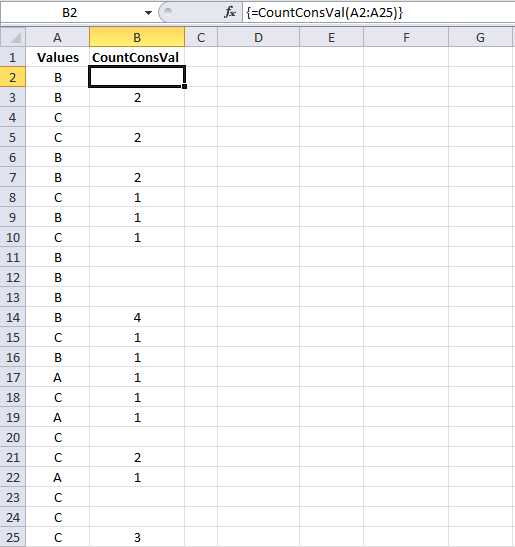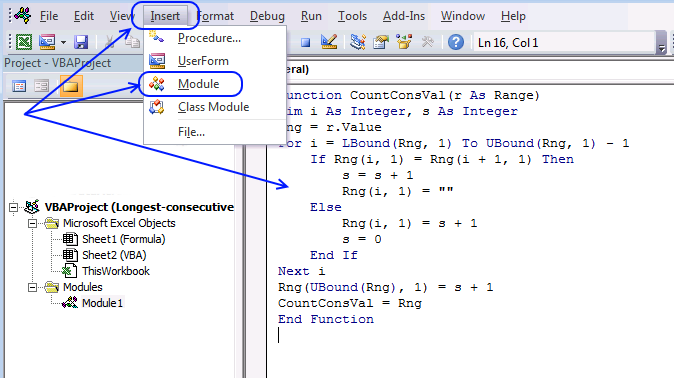Author: Oscar Cronquist Article last updated on March 24, 2021This post Find the longest/smallest consecutive sequence of a value has a few really big array formulas. Today I would like to show you how to build a simple UDF that will simplify these formulas significantly.

The following user-defined function returns the size of each consecutive sequence in a range. The udf is entered in cell range B2:B25, see this picture.

The UDF returns an array with the same size as the argument range. The cell range has 24 cells and the array has 24 values.

## 1. VBA code

```'Name User Defined Function
Function CountConsVal(r As Range)

'Dimension variables and declare data types
Dim i As Long, s As Long

'Save value in cell range r to variable Rng
Rng = r.Value

'Iterate through values in array variable Rng
For i = LBound(Rng, 1) To UBound(Rng, 1) - 1

'Check if next value is equal to current value
If Rng(i, 1) = Rng(i + 1, 1) Then

'Add 1 to numbers stored in variable s
s = s + 1

'Clear container in array variable Rng
Rng(i, 1) = ""

'Go here if next value is NOT equal to current value
Else

'Add 1 to variable s and save to container in array variable Rng
Rng(i, 1) = s + 1

'Save 0 to variable s
s = 0
End If
Next i

'Add 1 to variable s and then save result to the last container in array variable Rng
Rng(UBound(Rng), 1) = s + 1

'Return values to worksheet
CountConsVal = Rng
End Function

```

## 2. Array formulas

###Array formula in cell E3:

=MAX(CountConsVal(A2:A25))

Array formula in cell E4:

=INDEX(A2:A25,MATCH(MAX(CountConsVal(A2:A25)),CountConsVal(A2:A25),0))

Array formula in cell G3:

=MIN(CountConsVal(A2:A25))

Array formula in cell G4:

=INDEX(A2:A25,MATCH(MIN(CountConsVal(A2:A25)),CountConsVal(A2:A25),0))

Array formula in cell E7:

=LARGE(CountConsVal(A2:A25),2)

Array formula in cell E8:

=INDEX(A2:A25,MATCH(LARGE(CountConsVal(A2:A25),2),CountConsVal(A2:A25),0))

## 3. Where to put the code?

1. Press Alt + F11 to open the visual basic editor.
2. Press with left mouse button on "Insert" on the menu.
3. Press with left mouse button on "Module".4. Copy the code above and paste it to the code module.

## 4. Explaining the User Defined Function

#### 4.1 Function name and arguments

A user-defined function procedure always starts with "Function" and then a name. This UDF has a single argument r. Variable r is a range.

```Function CountConsVal(r As Range)
```

#### 4.2 Declaring variables

Variable i and s are declared data type Long. Read more about Defining data types.

```Dim i As Long, s As&nbsp;Long
```

#### 4.3 Transfer values from r (range) to a Rng (variant) array

```Rng = r.Value
```

#### 4.4 For ... Next statement

Repeats a group of statements a specified number of times. LBound returns the lower bound of an array and UBound the upper bound.

LBound(array, dimension) UBound(array, dimension), the dimension argument can be omitted if the array is a one-dimensional array. In this case the Variant Rng is a two-dimensional array despite the fact that the range r is a one-dimensional array. UBound(Rng, 1) returns the number of rows in Rng array.

```For i = LBound(Rng, 1) To UBound(Rng, 1) - 1

...

Next i
```

#### 4.5 If ... then... Else ... End If

Check if the current value (i) is equal to the next value (i+1)

```If Rng(i, 1) = Rng(i + 1, 1) Then
```

#### 4.6 Add number 1 to variable s

```s = s + 1
```

#### 4.7 Delete value in array

```Rng(i, 1) = ""
```

#### 4.8 Assign a value (s + 1) to array variable Rng(i, 1)

The current value in the array is equal to s + 1

```Rng(i, 1) = s + 1
```

```s = 0
```

#### 4.10 Assign a value (s + 1) to the last value in the array Rng(UBound(Rng), 1)

```Rng(UBound(Rng), 1) = s + 1
```

#### 4.11 The udf returns an array of values

```CountConsVal = Rng
```

#### 4.12 End a udf

A function procedure ends with this statement.

```End Function
```

### Get the Excel fileLongest-consecutive-sequence.xlsm